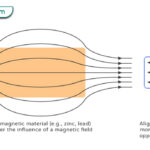Home / Physics / Can a Squirrel Survive Terminal Velocity?

# Can a Squirrel Survive Terminal Velocity?

Squirrels survive a fall because they have a low terminal velocity compared to other animals. The following equation gives the terminal velocity of an object falling through the air.

$v_{T} = \sqrt{\frac{2mg}{C\rho_{air} A}}$

A squirrel weighs 330 g, and the underside of its belly is approximately 500 cm2 in area. The drag coefficient for the belly down position is 1.

We have

m = 330 g = 0.33 kg

ρair = 1.29 kg/m3

C = 1

A = 500 cm2 = 0.05 m2

g = 9.81 m/s2

Therefore, the terminal velocity comes out to be

$v_T = \sqrt{\frac{2 \cdot 0.33 \hspace{1mm} kg \cdot 9.81\hspace{1mm} m/s^2}{1 \cdot 1.29\hspace{1mm} kg/m^3 \cdot 0.05\hspace{1mm} m^2}}\\ \Rightarrow v_T = 10 \hspace{1mm} m/s \hspace{1mm} \text{or} \hspace{1mm} 22.4 \hspace{1mm} mph$

This value is much lower than a skydiver, around 60 m/s. The reason for a low terminal velocity is that a squirrel has a sizeable area-to-mass ratio. Squirrels can spread their legs, increasing their body’s total surface and producing a “parachute effect”. This effect is further enhanced by their fur and large bushy tail. Therefore, the surface area of their body relative to their mass is reasonably large, reducing the aerodynamic drag. For this reason, the squirrel can resist the impact of that velocity and survive a fall.

Because it has a low terminal velocity, a squirrel achieves its terminal velocity during the first three seconds of its fall. It means that whether a squirrel falls from the top of a pine tree or a cliff, it will reach the ground at the same speed.

Article was last reviewed on Wednesday, June 22, 2022

### Related articlesWave-Particle DualityPhotoelectric EffectFerromagnetismDiamagnetism